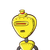# 25 km(v) A fraction becomes if 2 is added to both the numerator and the denominator.111, 3 is added to both the

25 km
(v) A fraction becomes if 2 is added to both the numerator and the denominator.
11
1, 3 is added to both the numerator and the denominator it becomes
5
Find the
fraction
6

### 1 thought on “25 km<br />(v) A fraction becomes if 2 is added to both the numerator and the denominator.<br />11<br />1, 3 is added to both the”

1.Let the fraction be

y

x

Given that

y+2

x+2

=

11

9

⇒11x+22=9y+18

⇒11x−9y+4=0→i

y+3

x+3

=

6

5

⇒6x+18=5y+15

⇒6x−5y+3=0→ii

⇒i×5−ii×9

⇒55x−45y+20=0

⇒54x−45y+27=0

eq(i)−eq(ii)

⇒x−7=0

⇒x=7

⇒y=

9

11x+4

=

9

11(7)+4

=

9

81

=9

∴Fraction is

y

x

=

9

7